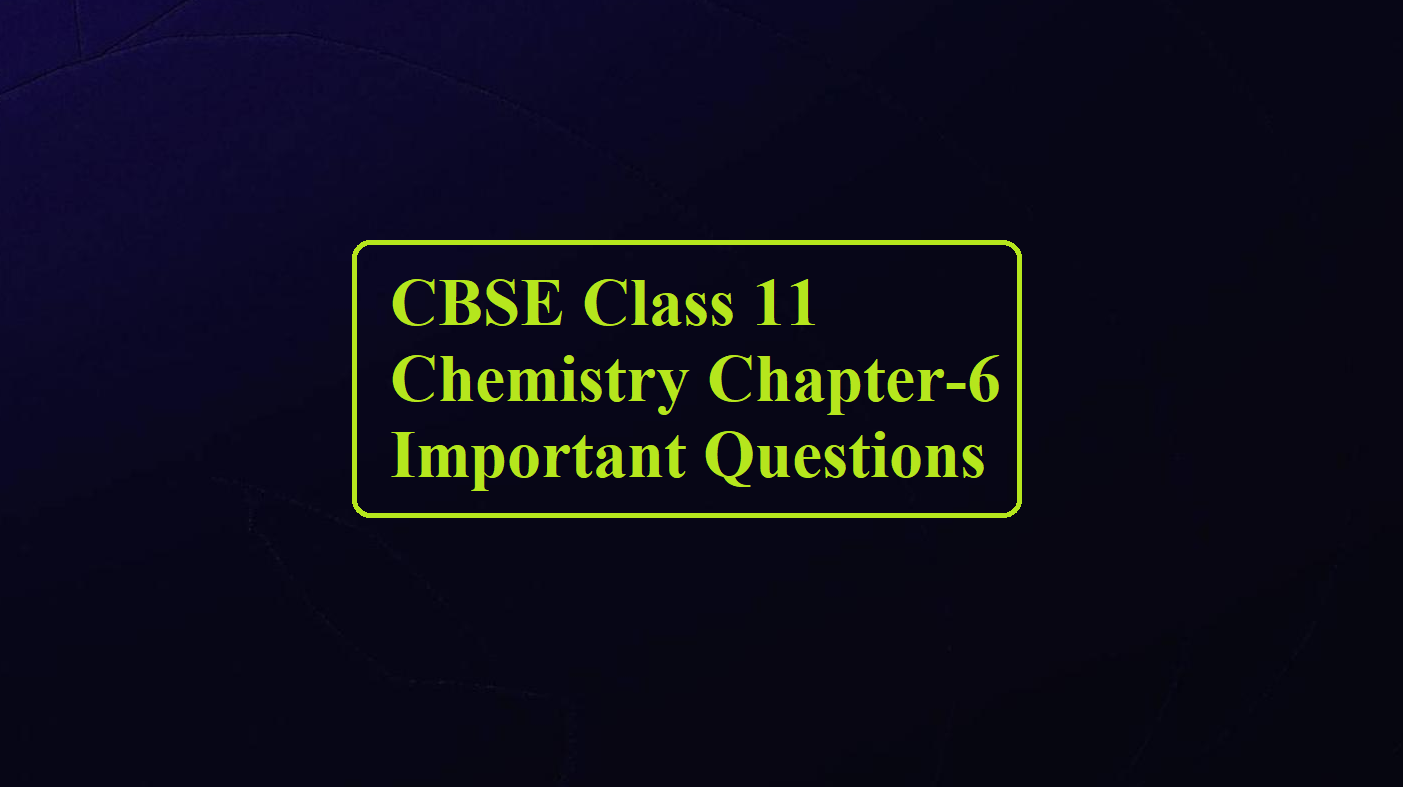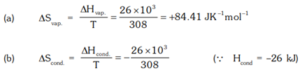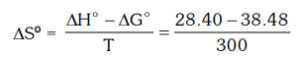# CBSE Class 11 Chemistry Chapter-6 Important Questions# CBSE Class 11 Chemistry Chapter-6 Important Questions

Class 11 Chemistry Chapter-6

Que 1. Neither q nor w is a state function but q + w is a state function. Explain.
Ans 1. Both q and W are not state functions but q + W is equal to ΔU which is a state function.

Que 2. A gas absorbs 200 J of heat and expands against the external pressure of 1.5 atm from a volume of 0.5 litres. Calculate the change in internal energy.
Ans 2. w = – PΔV = – 1.5 × (1.0 – 0.5) = – 0.75 litre atm
= – 0.75 × 101.3 J = – 75.975 J
1 litre atm = 101.3 J
Now, ΔE = 200 – 75.975 = + 124.025 J

Que 3. What is an adiabatic process?
Ans 3. A process during which no heat flows between the system and the surroundings is called an adiabatic process.

Que 4. 10 g of argon is compressed isothermally and reversibly at a temperature of 27°C from 10 L to 5 l. Calculate q, w, ∆U and, ∆H.
Ans 4. q = – 2.303 nRT log V2 / V1
= – 2.303 × 10/40 mol × 2 Cal K–1 mol–1 × 300 K × log 5/10 = –103.635 Cal
For isothermal compression ∆U = 0, W = ∆U – q = 0 – (– 103.635) = + 103.635 Cal
Also when temperature is constant, PV = constant, ∆H = ∆U + ∆(PV) = 0 + 0 = 0

Que 5. In the equation, N2 (g) + 3H2 (g) → 2NH3 (g). What would be the sign of work done?
Ans 5. The sing of work done will be positive, i.e., work will be done on the system due to decreases volumes.

Que 6. The enthalpy of vaporisation of liquid diethyl ether – (C2H5)2O, is 26.0 kJ mol–1 at its boiling point (35.0 0C). Calculate ΔS for conversion of
(a) liquid to vapour, and (b) vapour to liquid at 350C.
Ans 6.= – 84.41 JK–1 mol–1

Que 7. When a system absorbs a certain amount of heat and does work, how the change in internal energy can be thermodynamically related according to the first law of thermodynamics. Give the mathematical equation only.
Ans 7. ΔU = q + w, where w = negative as work is done by the system.

Que 8. What is spontaneous change? Give one example.
Ans 8. A process that can take place of its own or initiate under some condition. eg. Common salt dissolves in the water of its own.

Que 9. A gas expands by 1.5 litres against the constant pressure of one atmosphere. Calculate the work done in joule and calorie.
Ans 9. Work = – Pext × volume change
= – 1 × 1.5 = – 1.5 litre -atm = – 1.5 × 101.328 J = – 151.992 J
– 1.5 litre – atm = – 1.5 x 24.20 cal = – 36.30 cal.

Que 10. Calculate the free energy change when 1 mole of NaCl is dissolved in water at 25ºC. Lattice energy of NaCl = 777.8 kJ mol–1; ΔS for dissolution = 0.043 kJ mol–1 and hydration energy of NaCl =    –774.1 kJ mol–1.
Ans 10. ΔHdissolution = Lattice energy + Hydration energy
= 777.8 – 774.1 = 3.7 kJ mol–1
Now ΔG = ΔH – TΔS = 3.7 – 298 × 0.043
= 3.7 – 12.814
ΔG = – 9.114 kJ mol–1.

Que 11. Calculate the change in internal energy of the system when it absorbs 20 kJ of heat from the surroundings and does work equivalent to 50 kJ.
Ans 11. The change in internal energy is given by the expression ΔU = q + w
Here, q = 20 kJ w = – 50 kJ (negative sign implies the work is done by the system on the surrounding)
ΔU = 20 kJ + (– 50) kJ = 20 – 50 kJ = – 30 kJ
The internal energy of the system decreases by 30 kJ

Que 12. What is bond energy? Why is it called enthalpy of atomization?
Ans 12. It is the amount of energy required to dissociate one mole of bonds present b/w atoms in the gas phase. As molecules dissociate into atoms in the gas phase so bond energy of diatomic molecules is called enthalpy of atomization.

Que 13. Give the appropriate reason
(i) It is preferable to determine the change in enthalpy than the change in internal energy.
(ii) It is necessary to define the ‘standard state’.
(iii) It is necessary to specify the phases for the reactants and products in a thermochemical equation.
Ans 13. (i) Because it is easier to make measurements under constant pressure than under constant volume conditions.
(ii) Enthalpy changes depend upon the conditions in which a reaction is carried out. For making the comparison of results obtained by different people meaningful, the reactions conditions must be well-defined.
(iii) Because enthalpy depends upon the phase of reactants and products.

Que 14. The equilibrium constant for the reaction given below is 2.0 × 10–7 at 300 K. Calculate the standard free energy change for the reaction; PCl5(g) ↔ PCl3(g) + Cl2(g) Also, calculate the standard entropy change if ΔH0 = 28.40 kJ mol–1.
Ans 14. ΔG0 = – 2.303 × 8.314 × 300 log [2.0 × 10–7]
= +38479.8 J mol–1 = + 38.48 kJ mol–1
Also, ΔG0 = ΔH0 – TΔS0= – 0.0336 kJ = – 33.6 JK–1

Que 15. When an ideal gas expands into a vacuum, there is neither absorption nor evolution of heat. Why?
Ans 15. In an ideal gas, there are no intermolecular forces of attraction. Hence, no energy is required to overcome these forces. Moreover, when a gas expands against a vacuum, work done is zero (because pext = 0). Hence, the internal energy of the system does not change i.e., there is no absorption or evolution of heat.

Class 11 Chemistry Chapter-6